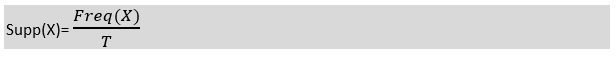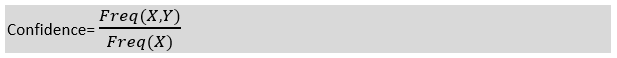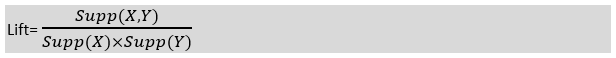# How Association rule mining works and Applications of Association rule mining/learning?

## How does Association Rule Learning work?

Association rule learning works on the concept of If and Else Statement, such as if A then B.Here the If element is called antecedent , and then statement is called as Consequent . These types of relationships where we can find out some association or relation between two items is known as single cardinality . It is all about creating rules, and if the number of items increases, then cardinality also increases accordingly. So, to measure the associations between thousands of data items, there are several metrics. These metrics are given below:

• Support
• Confidence
• Lift

Let’s understand each of them:

### Support

Support is the frequency of A or how frequently an item appears in the dataset. It is defined as the fraction of the transaction T that contains the itemset X. If there are X datasets, then for transactions T, it can be written as:### Confidence

Confidence indicates how often the rule has been found to be true. Or how often the items X and Y occur together in the dataset when the occurrence of X is already given. It is the ratio of the transaction that contains X and Y to the number of records that contain X.### Lift

It is the strength of any rule, which can be defined as below formula:It is the ratio of the observed support measure and expected support if X and Y are independent of each other. It has three possible values:

• If Lift= 1 : The probability of occurrence of antecedent and consequent is independent of each other.
• Lift>1 : It determines the degree to which the two itemsets are dependent to each other.
• Lift<1 : It tells us that one item is a substitute for other items, which means one item has a negative effect on another.

## Types of Association Rule Lerning

Association rule learning can be divided into three algorithms:

### Apriori Algorithm

This algorithm uses frequent datasets to generate association rules. It is designed to work on the databases that contain transactions. This algorithm uses a breadth-first search and Hash Tree to calculate the itemset efficiently.

It is mainly used for market basket analysis and helps to understand the products that can be bought together. It can also be used in the healthcare field to find drug reactions for patients.

### Eclat Algorithm

Eclat algorithm stands for Equivalence Class Transformation . This algorithm uses a depth-first search technique to find frequent itemsets in a transaction database. It performs faster execution than Apriori Algorithm.

### F-P Growth Algorithm

The F-P growth algorithm stands for Frequent Pattern , and it is the improved version of the Apriori Algorithm. It represents the database in the form of a tree structure that is known as a frequent pattern or tree. The purpose of this frequent tree is to extract the most frequent patterns.

## Applications of Association Rule Learning

It has various applications in machine learning and data mining. Below are some popular applications of association rule learning:

• Market Basket Analysis: It is one of the popular examples and applications of association rule mining. This technique is commonly used by big retailers to determine the association between items.
• Medical Diagnosis: With the help of association rules, patients can be cured easily, as it helps in identifying the probability of illness for a particular disease.
• Protein Sequence: The association rules help in determining the synthesis of artificial Proteins.
• It is also used for the Catalog Design and Loss-leader Analysis and many more other applications.